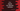# How to use logical not operator,! operator in C++## How to use logical not operator, ! operator in C++:

In this post, we will learn how to use logical not in C++. Logical not is used to reverse the operand state. If the operand is true, it will return false and vice versa.

In this post, we will learn how to use logical not in C++ with examples.

### Definition of logical not:

logical not is defined as like below:

``!operand``

or

``not(operand)``

The operand should give a boolean value. Logical not will reverse that value.

In C++, it will return 1 for true and 0 for false.

### Example of logical not:

Let’s take an example of logical not:

``````#include <iostream>

using namespace std;

int main()
{
int num = 3;

cout << !(num == 3) << endl;
cout << !(num != 3) << endl;
return 0;
}``````

It will print:

``````0
1``````

i.e. the first one is false and the second one is true.

We can also use not instead of !:

``````#include <iostream>

using namespace std;

int main()
{
int num = 3;

cout << not(num == 3) << endl;
cout << not(num != 3) << endl;
return 0;
}``````

It will give similar output.

### Example to take a user input number and check if it is even:

Let’s write one program that will take a number as input from the user and print out if it is even or not using logical not operator.

``````#include <iostream>

using namespace std;

int main()
{
int num;

cout << "Enter a number: " << endl;
cin >> num;

if (!(num % 2 == 0))
{
cout << "It is odd" << endl;
}
else
{
cout << "It is even" << endl;
}
return 0;
}``````

Here,

• num is an integer variable.
• It is reading one user input number and keep that in num
• The if condition checks the not value of num % 2 == 0. i.e. if num % 2 == 0 return true, it will be false. So, for even numbers, it will execute the else block and for odd numbers, it will execute the if block.

If you run this program, it will print output as like below:

``````Enter a number:
12
It is even

Enter a number:
13
It is odd``````